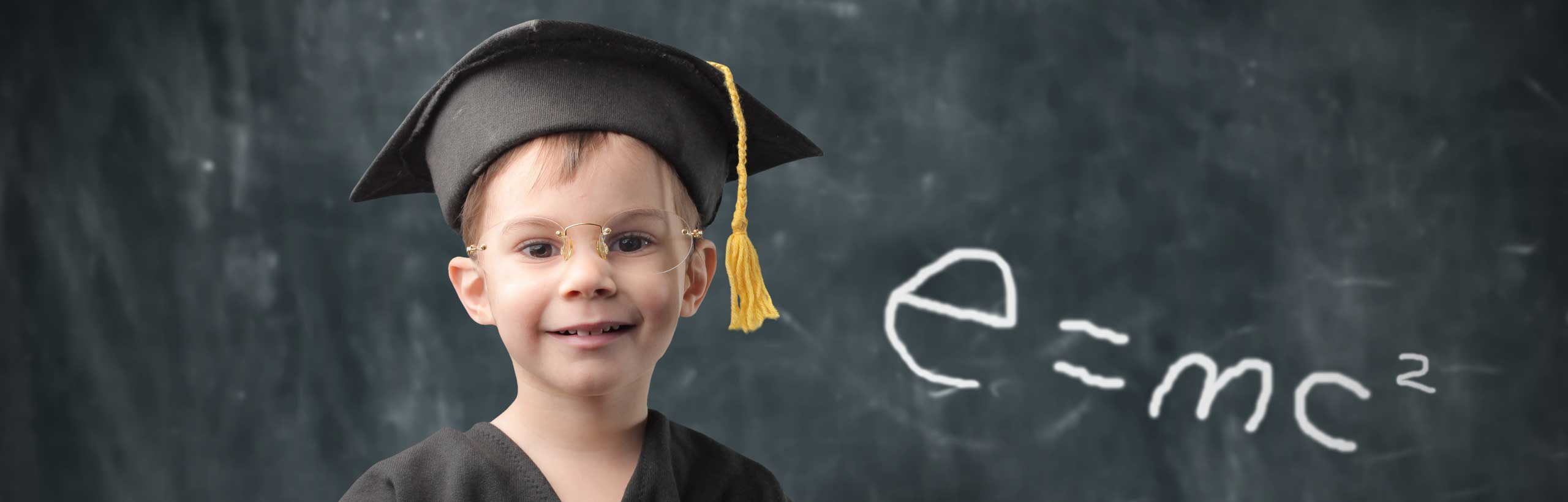# Solve First Order Differential Equations

A tutorial on how to solve first order differential equations. Examples with detailed solutions are included.

 The general form of the first order linear differential equation is as follows dy / dx + P(x) y = Q(x) where P(x) and Q(x) are functions of x. If we multiply all terms in the differential equation given above by an unknown function u(x), the equation becomes u(x) dy / dx + u(x) P(x) y = u(x) Q(x) The left hand side in the above equation has a term u dy / dx, we might think of writing the whole left hand side of the equation as d (u y ) / dx. Using the product rule of derivatives we obtain d (u y ) / dx = y du / dx + u dy / dx For y du / dx + u dy / dx and u(x) dy / dx + u(x) P(x) y to be equal, we need to have du / dx = u(x) P(x) Which may be written as (1/u) du / dx = P(x) Integrate both sides to obtain ln(u) = � P(x) dx Solve the above for u to obtain u(x) = e� P(x) dx u(x) is called the integrating factor. A solution for the unknown function u has been found. This will help in solving the differential equations. d(uy) / dx = u(x) Q(x) Integrate both sides to obtain u(x) y = � u(x) Q(x) dx Finally solve for y to obtain y = ( 1 / u(x) ) � u(x) Q(x) dx Example 1: Solve the differential equation dy / dx - 2 x y = x Solution to Example 1 Comparing the given differential equation with the general first order differential equation, we have P(x) = -2 x and Q(x) = x Let us now find the integrating factor u(x) u(x) = e� P(x) dx = e� -2 x dx = e - x2 We now substitute u(x)= e - x2 and Q(x) = x in the equation u(x) y = � u(x) Q(x) dx to obtain e - x2 y = � x e - x2dx Integrate the right hand term to obtain e - x2 y = -(1/2) e-x2 + C , C is a constant of integration. Solve the above for y to obtain y = C ex2 - 1/2 As a practice, find dy / dx and substitute y and dy / dx in the given equation to check that the solution found is correct. Example 2: Solve the differential equation dy / dx + y / x = - 2 for x > 0 Solution to Example 2 We first find P(x) and Q(x) P(x) = 1 / x and Q(x) = - 2 The integrating factor u(x) is given by u(x) = e� P(x) dx = e� (1 / x) dx = eln |x| = | x | = x since x > 0. We now substitute u(x)= x and Q(x) = - 2 in the equation u(x) y = � u(x) Q(x) dx to obtain x y = � -2 x dx Integrate the right hand term to obtain x y = -x2 + C , C is a constant of integration. Solve the above for y to obtain y = C / x - x As an exercise find dy / dx and substitute y and dy / dx in the given equation to check that the solution found is correct. Example 3: Solve the differential equation x dy / dx + y = - x3 for x > 0 Solution to Example 3 We first divide all terms of the equation by x to obtain dy / dx + y / x = - x2 We now find P(x) and Q(x) P(x) = 1 / x and Q(x) = - x2 The integrating factor u(x) is given by u(x) = e� P(x) dx = e� (1 / x) dx = eln |x| = | x | = x since x > 0. We now substitute u(x)= x and Q(x) = - x2 in the equation u(x) y = � u(x) Q(x) dx to obtain x y = � - x3 dx Integration of the right hand term yields x y = -x4 / 4 + C , C is a constant of integration. Solve the above for y to obtain y = C / x - x3 / 4 As an exercise find dy / dx and substitute y and dy / dx in the given equation to check that the solution found is correct. NOTE: If you can "see" that the right hand side of the given equation x dy / dx + y = - x3 can be written as d(x y) / dx, the solution can be found easily as follows d(x y) / dx = - x3 Integrate both sides to obtain x y = - x4 / 4 + C. Then solve for y to obtain y = - x3 / 4 + C / x Exercises: Solve the following differential equations. 1. dy / dx + y = 2x + 5 2. dy / dx + y = x4 Answers to Above Exercises 1. y = 2x + 3 + C e-x , C constant of integration. 2. y = x4 - 4x3 + 12x2 - 24 x + Ce-x + 24, C a constant of integration. More references on Differential Equations Differential Equations - Runge Kutta Method